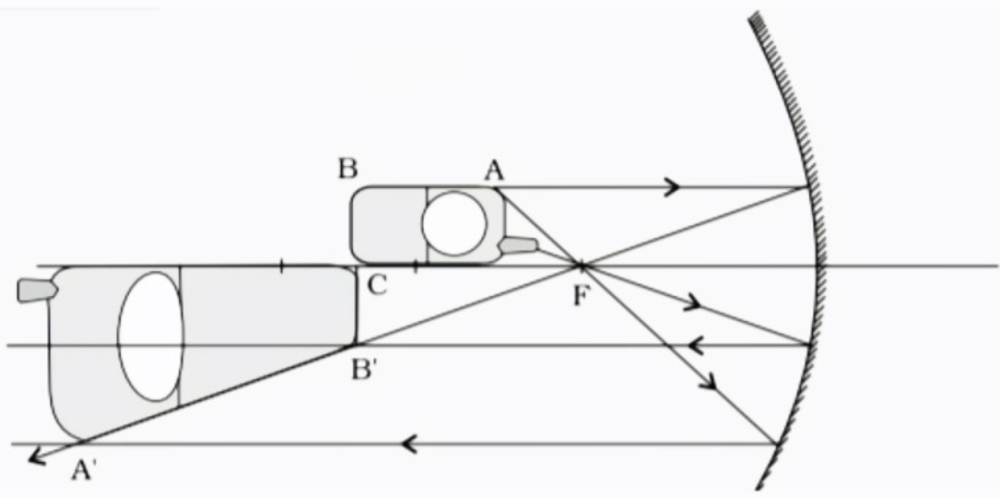Home/Class 12/Physics/

## QuestionPhysicsClass 12

A mobile phone lies along the principal axis of a concave mirror, as shown in figure. Show by suitable diagram, the formation of its image. Explain why the magnification is not uniform. Will the distortion of image depend on the location of the phone with respect to mirror ?

see analysis below
4.64.6## SolutionSolution -
The end $$A$$ of camera which acts like an object is placed between focus $$F$$ and centre of curvature$$C$$so concave mirror forms its image beyond centre of curvature and image is real as well as enlarged.
The end $$B$$of camera which acts like an object is placed at centre of curvature$$C$$ so concave forms its image at same point  i.e. at centre of curvature$$C$$and image is real and of same size as object  i.e. end$$B$$.
This is the reason why the magnification is not uniform.
Yes, distortion of image will depend on the location of the phone with respect to the mirror.